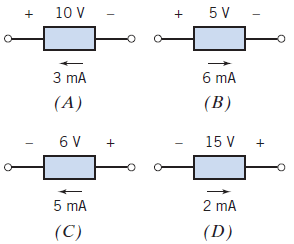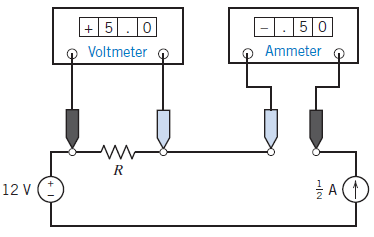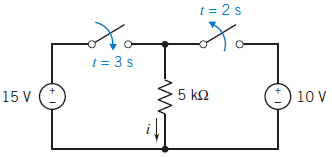# SSC JE Electrical Important MCQ Part 23

SSC JE Electrical Important MCQ PDF Part 23

1.Which of the devices supply 30 mWA. A & C

B. A & D

C. B & C

D. B & D

(Pa=10 V× 3 mA=30 mW

Pb=5 V× -6 mA=-30 mW

Pc=6 V× -5 mA=-30 mW

Pd=15 V× 2 mA=30 mW

It is clear from above devices A & D supply 30 mW and devices B & C absorb 30 mW)

2.Power absorbed by the VCVS in Figure isA. 6 W

B. 3 W

C. 4 W

D. 5 W

(The voltmeter measures vc to be vc=2 V

The voltage of the controlled voltage source is vd =2vc=4 V

The ammeter measures the current in the controlled voltage source to be id=1.5 A

Power absorbed by the voltage controlled-voltage source (VCVS) p= id×vd=1.5 × 4=6 W)

3.What is the value of the resistance RA. 5Ω

B. 10Ω

C. 15Ω

D. 20Ω

4.The value of current i at t=1 s for the circuit of Figure isA. 5 mA

B. 3 mA

C. 2 mA

D. 4 mA

(At t = 1 s the left switch is open and the right switch is closed so the voltage across the resistor is 10 V.

i=v/R=10/5 kΩ=10/5×10³=2 mA)

Note-Trick for switch problems5.What is the resistance R in Figure so that v > 40 VA. R > 20 Ω

B. R=0 Ω

C. R< 20 Ω

D. R > 10 Ω

Answer : A (V=IR⇒2R > 40 ⇒ R > 20 Ω)

6.A generating station has a maximum demand of 35 MW and has a connected load of 60 MW.What will be the demand factor

A. 583 %

B. 5.83 %

C. 0.583 %

D. 58.3 %

7.A generating station supplies four feeders with the maximum demands (in MW) of 16, 10, 12, and 7 MW. The overall maximum demand on the station is 20 MW and the annual load factor is 45%. What will be the diversity factor

A. 2.25

B. 22.5

C. 255

D. 0.225

(Sum of maximum demands = 16 + 10 + 12 + 7 = 45 MW

Simultaneous maximum demand = 20 MW

∴ Diversity factor=Sum of maximum demands/Simultaneous maximum demand=45/20=2.25)

8.What is the total annual energy generated, if the maximum demand on a power station is 120 MW and the annual load factor is 50%.

A. 525.6 × 10³ kWh

B. 525.6 × 10⁶ kWh

C. 52.56 × 10³ kWh

D. 52.56 × 10⁶ kWh

(Maximum demand on a power station = 120 MW

Load factor =(Energy generated/annum) /maximum demand × hours in a year

Energy generated/annum= maximum demand × Load factor × hours in a year

= (120 × 10³) × (0.5) × (24 × 365) kWh

= 525.6 × 10⁶ kWh)

9.The maximum demand on a generating station is 20 MW, a load factor of 75%, a plant capacity factor of 50%, and a plant-use factor of 80%.Reserve capacity of the plant is

A. 20 MW

B. 30 MW

C. 40 MW

D. 10 MW

(Maximum demand,MD= 20 MW

Average load = MD × LF = 20 × 0.75 = 15 MW

Plant capacity factor = 50%=0.5

Power station installed capacity =Average load/Plant capacity factor=15/0.5=30 MW

Plant reserve capacity = installed capacity-maximum demand = 30 − 20= 10 MW)

10.A consumer has a connected load of 12 lamps each of 100 W at his/ her premises. His/ her load demand is as follows
From midnight to 5 A.M.: 200 W.
5 A.M. to 6 P.M.: no load.
6 P.M. to 7 P.M.: 700 W.
7 P.M. to 9 P.M.: 1,000 W.
9 P.M. to midnight: 500 W.

A. 216.7 W

B. 21.67 W

C. 2.167 W

D. 216.6 MW

(Electrical energy consumption during the day= 200 × 5 + 700 × 1 + 1,000 × 2 + 500 × 3= 5,200 Wh

Average load=energy consumption during the day/24=5200/24=216.7 W)

11.A diesel power station has fuel consumption 0.2 kg per kWh. If the calorific value of the oil is 11,000 kcal per kg.Then the overall efficiency of the power station is

A. 3.92%.

B. 39.2%.

C. 392%.

D. 0.392%.

(For 1 kWh output, Heat input = 11,000 × 0.2 = 2200 kcal.

Now 1 kWh = 866 kcal. So Overall efficiency =Output/Input=866/2200= 39.2%)

12.For electroplating power, which of the following generators should be used

A. Separately excited

B. Delta system

C. Split phase

D. None of these

Answer : A (Separately excited generator-It is used for electroplating and booster. It can be used for lighting and power purpose with field regulator.)

13.In a generator, voltage is produced by which of the following conditions

A. Electrical pressure

B. Vibration

C. Magnetism

D. Cutting lines of force

14.Illumination is measured in which of the following units

A. Lumens

B. Foot candles

C. Light per square inch

D. None of these

Answer : B (Foot-candle is a non-SI unit of illuminance or light intensity. The foot-candle is defined as one lumen per square foot)

15.Which of the following terms is used to describe the inductive action, which causes current to flow on the outer surface of a conductor

A. Halo effect

B. Inductance

C. Skin effect

D. Insulated par value

16.Two transmission wires create corona when which of the following exists

A. The wires have a high potential difference

B. The wires are installed overlapping or too close together

C. The wires are spaced too far apart

D. None of these

17.If a series circuit has unequal resistances throughout the circuit, then which of the following is true

A. The lowest resistance has the highest current.

B. The lowest resistance has the highest voltage.

C. The highest resistance has the highest voltage.

D. The highest resistance has the highest current.

Answer : C (In a series circuit current I is same,So V ⋉ R)

18.The output of a 3-phase transformer is measured in which of the following units

A. Volts

B. Amperes

C. Volt-amps

D. Watts

Answer : C (Single Phase & 3 Phase Transformer always rated in kVA)

19.In a coil, the higher the level of self-inductance

A. The lower the level of resistance will be

B. The longer the delay will be in establishing current through it

C. The greater the level of flux produced will be

D. None of these

20.If the power factor of a circuit is increased, then which of the following occurs

A. The active power is increased

B. The reactive power is decreased

C. Both of these

D. None of these